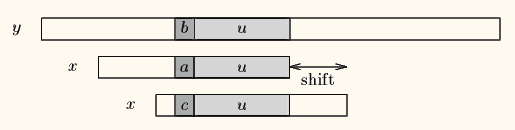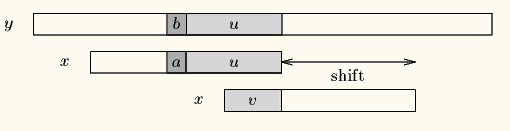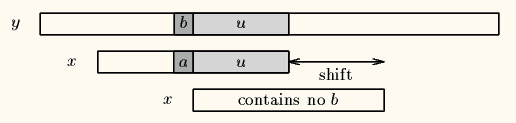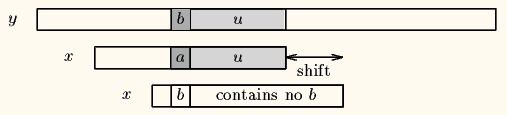# Boyer-Moore Algorithm

String is an inevitable part in programming. In large sphere, it is essential element in computer science. Searching for a specific string or matching a string is one of the common task in computer science related work. I will discuss about one of the string searching algorithm - Boyer-Moore algorithm.

### Boyer-Moore Algorithm

Boyer-Moore algorithm, like Knuth-Morris-Pratt or Rabin-Karp algorithm, is a string searching algorithm which is the standard benchmark for efficient string-search practise. it was developed by Robert Boyer and J Strother Moore in 1977. This algorithm preprocesses the pattern, but not the the test string. It is thus well-suited for applications in which the pattern is much shorter than the text. The Boyer-Moore algorithm runs faster as the pattern length increases. The key features of the algorithm are to start matching from the tail of the pattern rather than the head, and skipping the text for multiple characters rather than searching every character in the text. A simplified version of it or the entire algorithm is often implemented in text editors for the «search» and «substitute» commands.

### Algorithm

Like the arabic sentence, this algorithm scans the characters of the pattern from right to left beginning with the rightmost one. In case of a mismatch (or a complete match of the whole pattern) it uses two precomputed functions to shift the window to the right. These two shift functions are called the good-suffix shift (also called matching shift) and the bad-character shift (also called the occurrence shift). It preporcesses the pattern and creates different arrays for both heuristics. At every step, it slides the pattern by max of the slides suggested by the two heuristics. So it uses best of the two heuristics at every step.

Let us think that a mismatch occurs between the character x(i)=a of the pattern and the character y(i+j)=b of the text during an attempt of matching at position j.Then, x(i+1 .. m-1)=y(i+j+1 .. j+m-1)=u and x(i) neq y(i+j). The good-suffix shift consists in aligning the segment y(i+j+1 .. j+m-1)=x(i+1 .. m-1) with its rightmost occurrence in x that is preceded by a character different from x(i)If there exists no such segment, the shift consists in aligning the longest suffix v of y(i+j+1 .. j+m-1) with a matching prefix of x.The bad-character shift consists in aligning the text character y(i+j) with its rightmost occurrence in x(0 .. m-2).If y(i+j) does not occur in the pattern x, no occurrence of x in y can include y(i+j), and the left end of the window is aligned with the character immediately after y(i+j), namely y(i+j+1)

### Implementation

The ruby implementation of boyer-moore algorithm is written below

``````def pre_bad_character x, m
bmBc = Array.new 256
a_size = 256
(0...a_size).to_a.each do |i|
bmBc[i] = m
end
(0...m-1).to_a.each do |i|
bmBc[x[i]] = m - i - 1
end
end

def suffixes x, m, suff
suff[m - 1] = m
g = m - 1
i = m - 2
f = 0
until i >= 0 do
next suff[i] = suff[i + m - 1 - f] if i > g && suff[i + m - 1 - f] < i - g
g = i if i < g
f = i
until g >= 0 && x[g] == x[g + m - 1 - f] do
g -= 1
end
suff[i] = f - g
end
suff
end

def pre_good_suffix x, m
bmGs = Array.new x.length
suff = Array.new x.length
suff = suffixes x, m, suff
(0...m).to_a.each do |i|
bmGs[i] = m
end
j = 0;
i = m-1
until i >= 0 do
if suff[i] == i + 1
until j < m - 1 do
bmGs[j] = m - 1 - i if bmGs[j] == m
j += 1
end
end
i += 1
end
(0..m-2).to_a.each do |i|
bmGs[m - 1 - suff[i]] = m - 1 - i
end
bmGs
end

def boyer_moore p, m, t, n) {
bmGs = preBmGs p, m
bmBc = preBmBc p, m
j = 0
until j <= n - m do
i = m - 1
until i >= 0 && x[i] == y[i + j]
i -= 1
end
if i < 0 do
print j
j += bmGs
else
j += [bmGs[i], bmBc[y[i + j]] - m + 1 + i].max
end
end
end
``````

### Complexity

Table bad character and good character can be pre-computed in O(m + q), where q is the length of two tables.This algorithm may take O(mn) time in worst case. The worst case occurs when all characters of the text and pattern are same. For example, T = “AAAAAAAAAAAAAAAAAA” and p = “AAAAA”. The best case complexity is O(m/n). For large alphabet pattern this algorithm works faster. O(m/n) is better that the best case performance of other string processing algorithms.

Thank you